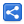# TypeScript - Type Inference

[Last Updated: Sep 4, 2018]

In this tutorial we will understand, on basic level, how types are inferred in TypeScript.

In TypeScript, the compiler can infer the type information if no explicit type has been specified. The inference is done based on the assigned value.

The type inference takes place when initializing variables and members, setting parameter default values, and determining function return types.

## Variable initialization

`let count = 5;`

In above snippet 'count' type has been inferred as number. That's because of the assigned value; the compiler can see that right side value 5 is a number. After inference, the compiler sees above line as let count: number = 5;
Now if we try to perform anything on 'count' which is not compatible with number, the compiler will throw an error:

```let count = 5;
let result = count.substring(0, 2);//error thrown here

```
`D:\typescript-type-inference>tsc example1.tsexample1.ts(2,20): error TS2339: Property 'substring' does not exist on type 'number'.`

IDEs can still help us in pointing out the errors on inferred types: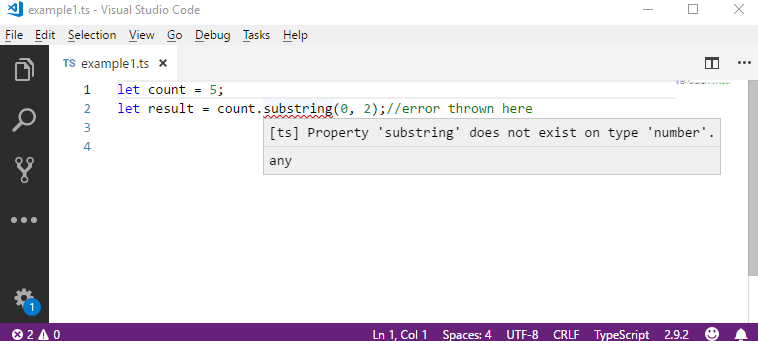## Function return type

Following example shows how type is inferred by the compiler in case of a function return type:

```function getSomething() {
return 3;//inference based on return value, type is number;
}

let result = getSomething();
let len = result.substring(0,2);//error thrown here```
`D:\typescript-type-inference>tsc example2.tsexample2.ts(6,18): error TS2339: Property 'substring' does not exist on type 'number'.`

## Default parameters

Following example shows how types are inferred from function default parameters:

```function multiply(b = 1) {
b.substring(0, 2);
}```
`D:\typescript-type-inference>tsc example3.tsexample3.ts(2,7): error TS2339: Property 'substring' does not exist on type 'number'.`

## Example Project

Dependencies and Technologies Used:

• TypeScript 3.0.1
 TypeScript - Type Inference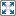Select All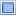Download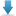• typescript-type-inference
• example1.ts

 Share